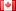# Revision history [back]

### Importing file to Quadratic field

I have a file with a list of elements inside the square of a Quadratic field, i.e. QQ(sqrt)^2. The file looks like [[2 + sqrt,4 - 2*sqrt],[2,sqrt],...]. I want to import this file into a single matrix over the field, in the previous example F. = QuadraticField(13). Currently, I read everything in as a string then convert to the matrix, but there must be an easier way to do this. I am somewhat new to python programming in general.2 improved stylefidbc2198 ●4 ●21 ●51

### Importing file to Quadratic field

I have a file with a list of elements inside the square of a Quadratic field, i.e. QQ(sqrt)^2. QQ(sqrt)^2. The file looks like [[2 + sqrt,4 - 2*sqrt],[2,sqrt],...]. 2*sqrt],[2,sqrt],...]. I want to import this file into a single matrix over the field, in the previous example F. F.<a> = QuadraticField(13). QuadraticField(13). Currently, I read everything in as a string then convert to the matrix, but there must be an easier way to do this. I am somewhat new to python programming in general.3 clearer title

### Importing Convert a text file to Quadratic fieldinto a matrix

I have a file with a list of elements inside the square of a Quadratic field, i.e. QQ(sqrt)^2. The file looks like [[2 + sqrt,4 - 2*sqrt],[2,sqrt],...]. I want to import this file into a single matrix over the field, in the previous example F.<a> = QuadraticField(13). Currently, I read everything in as a string then convert to the matrix, but there must be an easier way to do this. I am somewhat new to python programming in general.4 retaggedFrédéricC2364 ●3 ●27 ●47

### Convert a text file into a matrix

I have a file with a list of elements inside the square of a Quadratic field, i.e. QQ(sqrt)^2. The file looks like [[2 + sqrt,4 - 2*sqrt],[2,sqrt],...]. I want to import this file into a single matrix over the field, in the previous example F.<a> = QuadraticField(13). Currently, I read everything in as a string then convert to the matrix, but there must be an easier way to do this. I am somewhat new to python programming in general.